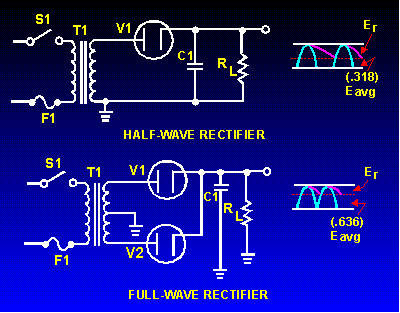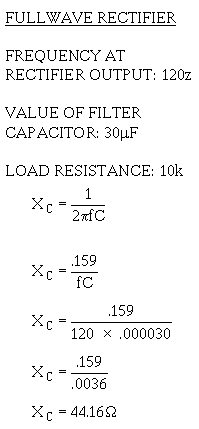power supply filter. The use of this filter is very limited. It is sometimes used on extremely high-voltage, low-current power supplies for cathode-ray and similar electron tubes that require very little load current from the supply. This filter is also used in circuits where the power-supply ripple frequency is not critical and can be relatively high. ">Custom SearchThe Capacitor Filter The simple capacitor filter is the most basic type of power supply filter. The use of this filter is very limited. It is sometimes used on extremely high-voltage, low-current power supplies for cathode-ray and similar electron tubes that require very little load current from the supply. This filter is also used in circuits where the power-supply ripple frequency is not critical and can be relatively high. The simple capacitor filter shown in figure 3-20 consists of a single-filter element. This capacitor (C1) is connected across the output of the rectifier in parallel with the load. The RC charge time of the filter capacitor (C1) must be short and the RC discharge time must be long to eliminate ripple action when using this filter. In other words, the capacitor must charge up fast with preferably no discharge at all. Better filtering also results when the frequency is high; therefore, the full-wave rectifier output is easier to filter than the half-wave rectifier because of its higher frequency. Figure 3-20. - Full-wave rectifier with a capacitor filter.To understand better the effect that filtering has on E avg , compare the rectifier circuits without filters in figure 3-21 to those with filters in figure 3-22. The output waveforms in figure 3-21 represent the unfiltered outputs of the half-wave and full-wave rectifier circuits. Current pulses flow through the load resistance (RL) each time a diode conducts. The dashed line indicates the average value of output voltage. For the half-wave rectifier, Eavg is less than half the peak output voltage (or approximately 0.318 of the peak output voltage). For the full-wave rectifier, Eavg is approximately 0.637. This value is still much less than the applied voltage. With no capacitor connected across the output of the rectifier circuit, the waveform has a large pulsating component (ripple) compared with the average or dc component. Now refer to figure 3-22. When a capacitor is connected across the output (in parallel with RL), the average value of output voltage (Eavg) is increased due to the filtering action of capacitor C1. Figure 3-21. - Half-wave/full-wave rectifiers (without filters).Figure 3-22. - Half-wave/full-wave rectifiers (with capacitor filters).The value of the capacitor is fairly large (several microfarads); it thus presents a relatively low reactance to the pulsating current and stores a substantial charge. The rate of charge for the capacitor is limited only by the relatively low resistance of the conducting diode. The RC charge time of the circuit is, therefore, relatively short. As a result, when the pulsating voltage is first applied to the circuit, the capacitor charges rapidly and almost reaches the peak value of the rectified voltage within the first few cycles. The capacitor attempts to charge to the peak value of the rectified voltage anytime a diode is conducting, and tends to retain its charge when the rectifier output falls to zero. (The capacitor cannot discharge immediately). The capacitor slowly discharges through the load resistance (RL) during the time the rectifier is nonconducting. The rate of discharge of the capacitor is determined by the value of capacitance and the value of the load resistance. If the capacitance and load resistance values are large, the RC discharge time for the circuit is relatively long. From the waveforms shown in figure 3-22, you should see that the addition of C1 to the circuit results in an increase in the average value of output voltage (Eavg) and a reduction in the amplitude of the ripple component (Er) present across the load resistance. Now, let's consider a complete cycle of operation using a half-wave rectifier, a capacitive filter (C1), and a load resistor (RL). As shown in figure 3-23, C1 is assumed to be large enough to ensure a small reactance to the pulsating rectified current. The resistance of RL is assumed to be much greater than the reactance of C1 at the input frequency. Figure 3-23. - Half-wave rectifier capacitor filter (positive input cycle).When the circuit is energized, the diode conducts on the positive half cycle and current flows through the circuit allowing C1 to charge. C1 will charge to approximately the peak value of the input voltage. The charge is less than the peak value because of the voltage drop across diode V1. The charge on C1 is indicated by the heavy, solid line on the waveform. As illustrated in figure 3-24, the diode (V1) cannot conduct on the negative half cycle because the plate of V1 is negative in respect to the cathode. During this interval, C1 discharges through load resistance RL. The discharge of C1 produces the downward slope indicated by the solid line on the waveform in the figure. Figure 3-24. - Half-wave rectifier capacitor filter (negative input cycle).During the discharge period, in contrast to the abrupt fall of the applied ac voltage from peak value to zero, the voltage across C1 (and thus across RL) gradually decreases until the time of the next half cycle of rectifier operation. Keep in mind that for good filtering, the filter capacitor should charge as fast as possible and discharge as little as possible. Since practical values of C1 and RL ensure a more or less gradual decrease of the discharge voltage, a substantial charge remains on the capacitor at the time of the next half cycle of operation. As a result, no current can flow through the diode until the rising ac input voltage at the plate of the diode exceeds the voltage of the charge remaining on C1. The charge on C1 is the cathode potential of the diode. When the potential on the plate exceeds the potential on the cathode (the charge on C1), the diode again conducts, and C1 commences to charge to approximately the peak value of the applied voltage. After the capacitor has charged to its peak value, it begins to discharge. Since the fall of the ac input voltage on the plate is considerably more rapid than the decrease on the capacitor voltage, the cathode quickly becomes more positive than the plate, and the diode ceases to conduct. The operation of the simple capacitor filter using a full-wave rectifier is basically the same as the operation we discussed for the half-wave rectifier. Notice in figure 3-25 that because one of the diodes is always conducting on either alternation, the filter capacitor charges and discharges during each half cycle. (Note that each diode conducts only for that portion of time when the peak secondary voltage is greater than the charge across the capacitor.) Figure 3-25. - Full-wave rectifier (with capacitor filter).We stated before that a major advantage of full-wave and bridge rectifiers over half-wave rectifiers is the ease of filtering their output voltages. You can now see the reason for this. The ripple frequency is doubled; therefore, the time period the capacitor is allowed to discharge is cut in half. This means that the capacitor discharges less. Thus, ripple amplitude is less, and a smoother output voltage occurs. Another thing to keep in mind is that the ripple component (E r) of the output voltage is an ac voltage and the average output voltage (Eavg) is the dc component of the output. Since the filter capacitor offers a relatively low impedance to ac, the majority of the ac component flows through the filter capacitor. The ac component is therefore bypassed (shunted) around the load resistance and the entire dc component (or Eavg) flows through the load resistance. To clarify this statement, let's take a look at the formula for XC in a half-wave and full-wave rectifier. First, you must establish some values for the circuit.As you can see from the calculations, when the output frequency of the rectifier is doubled, the impedance of the capacitor is reduced by one-half. Therefore, when the simple capacitor filter is used in conjunction with a full-wave or bridge rectifier, improved filtering is provided because the increased ripple frequency decreases the capacitive reactance of the filter capacitor. This allows the ac component to be passed through the capacitor more easily. Therefore, the output of a full-wave rectifier is much easier to filter than that of a half-wave rectifier. It should be obvious that the smaller the XC of the filter capacitor in respect to the load resistance, the better the filtering action. By using the largest possible capacitor, we achieve the best filtering. The load resistance is also an important consideration. If load resistance is made small, the load current increases, and the average value of output voltage (Eavg) decreases. The RC discharge time constant is a direct function of the value of the load resistance; therefore, the rate of capacitor voltage discharge is a direct function of the current through the load. The greater the load current, the more rapid the discharge of the capacitor, and the lower the average value of output voltage. For this reason, the simple capacitor filter is seldom used with rectifier circuits that must supply a relatively large load current. Q.17 What is the most basic type of filter?Q.18 In a capacitor filter, is the capacitor in series or parallel with the load?Q.19 Is better filtering achieved at a high frequency or at a low frequency at the input of the filter?Q.20 Does a filter circuit increase or decrease the average output voltage?Q.21 What determines the rate of discharge of the capacitor in a filter circuit?Q.22 Does low ripple voltage indicate good or bad filtering?Q.23 Is a full-wave rectifier output easier to filter than that of a half-wave rectifier?Integrated Publishing, Inc. - A (SDVOSB) Service Disabled Veteran Owned Small Business